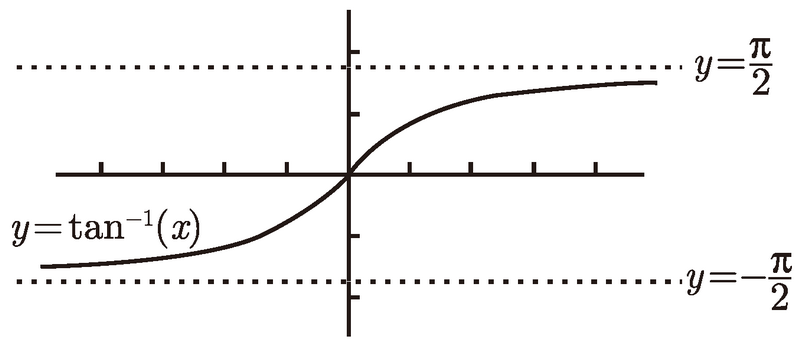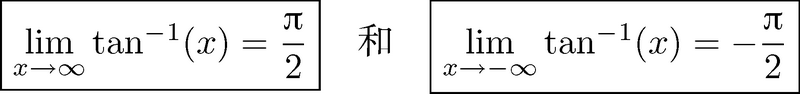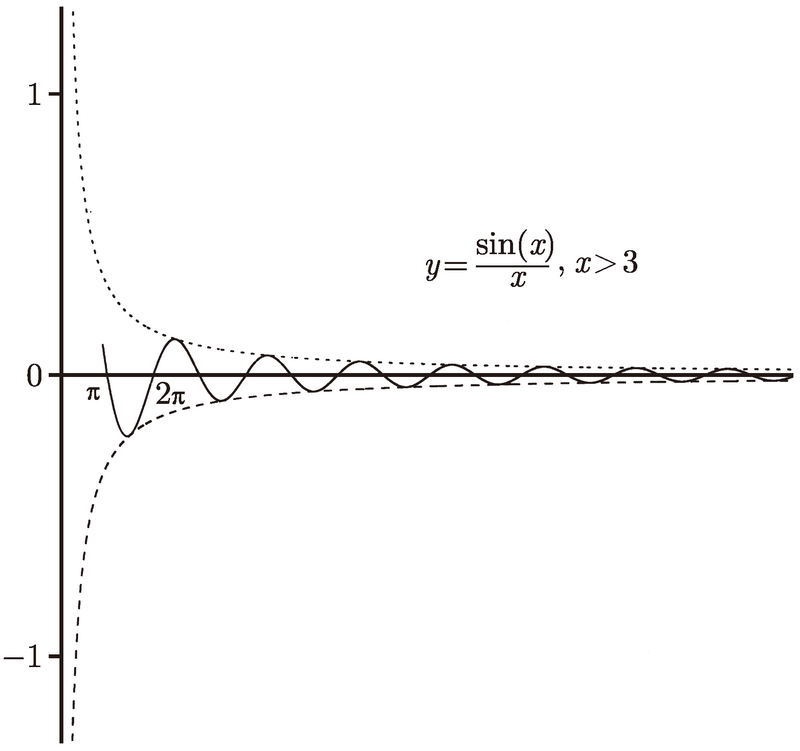# 普林斯顿微积分读本（修订版）(25)：极限导论 3.5(关于渐近线的两个常见误解)

$\lim_{x\to\infty}\frac{1}{x}=0$　和　$\lim_{x\to-\infty}-\frac{1}{x}=0$.$\lim_{x\to\infty}\frac{\sin(x)}{x}=0$